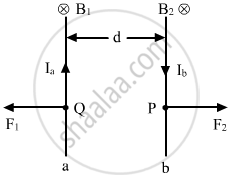Share

# Show with the Help of a Diagram How the Force Between the Two Conductors Would Change When the Currents in Them Flow in the Opposite Directions? - Physics

ConceptMotion in a Magnetic Field

#### Question

Show with the help of a diagram how the force between the two conductors would change when the currents in them flow in the opposite directions?

#### SolutionNow, let the direction of current in conductor b be reversed. The magnetic field B2 at point P due to current Ia flowing through a will be downwards. Similarly, the magnetic field B1 at point Q due to current Ib passing through b will also be downwards as shown. The force on a will be, therefore, towards the left. Also, the force on b will be towards the right. Hence, the two conductors will repel each other as shown.

Is there an error in this question or solution?

#### APPEARS IN

Solution Show with the Help of a Diagram How the Force Between the Two Conductors Would Change When the Currents in Them Flow in the Opposite Directions? Concept: Motion in a Magnetic Field.
S